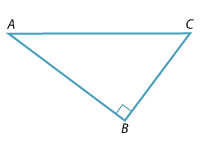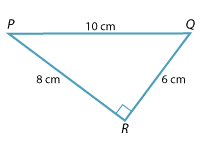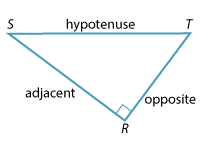#### Question 1

Consider the triangle $$ABC$$ pictured below.Using the reference angle $$\angle BCA$$

 a b c The opposite side is? $$AB$$ $$BC$$ $$CA$$ The adjacent side is? $$AB$$ $$BC$$ $$CA$$ The hypotenuse is? $$AB$$ $$BC$$ $$CA$$

#### Question 2

Consider the triangle PQR pictured below.Detailed description

Using the reference angle $$\angle QPR$$

 a b c The length of the opposite side is? 6 cm 8 cm 10 cm The length of the adjacent side is? 6 cm 8 cm 10 cm The length of the hypotenuse is? 6 cm 8 cm 10 cm

#### Question 3

Consider the triangle $$RST$$ below.Detailed description

The angle which has been used as the reference angle for the naming of the sides is:

 $$\angle RST$$ $$\angle TSR$$ $$\angle SRT$$